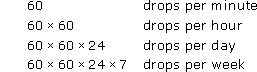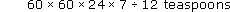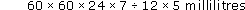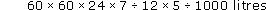Tapping into mathematics

Start this free course now. Just create an account and sign in. Enrol and complete the course for a free statement of participation or digital badge if available.

Free course

# 5.8 Nor any drop to drink

## Exercise 9

On the basis of the figures and assumptions given in Activity 8, calculate the following, using your calculator where appropriate.

• (a) How many litres of water are lost in one week?

• (b) How many baths or buckets is this?

• (c) Compare the costs of employing a nurse for a week with the cost of the water wasted.

• (d) How likely does the politician's claim seem now?

• (a) If the tap drips once per second then there will be

•• With 12 drops per teaspoon this is

•• Each teaspoon is 5 millilitres, so this is

•• Since 1000 millilitres = 1 litre, this is

•• Entering this on the calculator gives an approximate figure of 252 litres of water wasted in a week.

• (b) Since one cubic metre of water (or 10000 litres) of water is approximately 90 buckets full, and since 252 litres is about a quarter of this it seems that the dripping tap wastes about 90 ÷ 4 buckets or about 23 buckets full per week.

• Alternatively, since an average bath uses 90 litres, this is the same as 252 ÷ 90 baths. That is about 3 average baths or probably about one bath full to the brim.

• (c) The weekly cost of the nurse will be £15,000 ÷ 52, which is about £290 per week.

• The hospital pays about £0.91 per cubic metre for water, so the dripping tap costs the hospital roughly

•   252 ÷ 1000 × £0.91 = £0.23 per week.

• (d) On the basis of these calculations the claim looks pretty suspect!

• The cost of the dripping tap is less than a thousandth of the cost of employing a nurse.

Of course, we made some assumptions in reaching this conclusion. First, we assumed a drip rate of one per second. Let us be daring now and make the assumption that the tap is dripping at a rate of 10 drips per second. Using this assumption, the weekly cost of the water you have just calculated should be multiplied by 10.

Also, our method of measurement was, at best, rather rough and ready. However, if we had decided that it took only, say, six drops rather than 12 to fill a teaspoon, the cost of the water would still only be doubled.

We also assumed that the water wasted was cold. But even if the hospital had been losing water which it had paid to heat, it is still unlikely that one bath (or even 10 baths or 20 baths) full of hot water would cost anything like the cost of employing a nurse.

The point of the calculation was not to produce an exact answer – the accuracy of the input data we used would not warrant that – but rather to check whether the order of magnitude of the politician's claim was reasonable. The results suggest that the claim does not stand up to investigation. Our check did not require much in the way of complicated mathematics, just a few facts and figures and one or two fairly straightforward calculations.

Next time you come across similar claims don't automatically assume that they are true. Do your own short investigation and check them out for yourself, with your mathematical tool (the calculator) ready at hand.

MU120_4MCAL